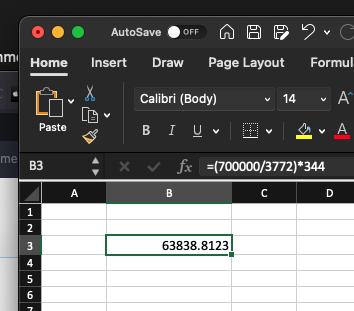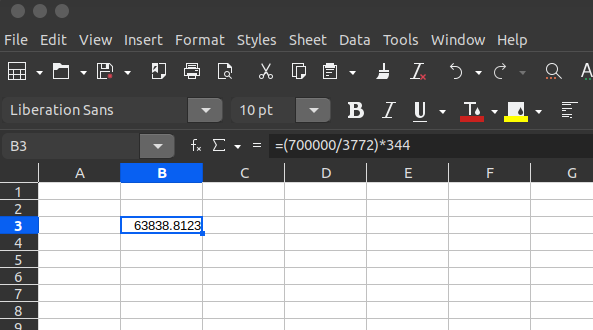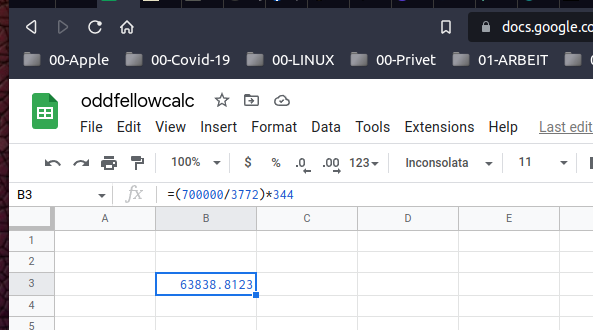# Calculation error in LibreOffice Calc and MS Excel

Dear Learned Members,

I have a following problem. I am filing my annual income tax return and for which I am calculating my income. The following is the formula and its result in LibreOffice Calc and MS Excel :

(700000/3772)*344 = 63844.45

The same formula when I write in LibreOffice Writer using calculation function, gives me the following result :

(700000/3772)*344 = 63838.81

The value given by LibreOffice Writer is the correct one.

While I am not able to figure out why this error, my real issue is, even a small increase in my income, will put me in next slab of income tax.

Request for valuable input for achieving the correct value in LibreOffice Calc.

Hi @ramki_1965,
Using LiberOffice Calc Version 6.4.7.2, I got the correct answer.
I used “=SUM((700000/3772)*344)”

Hi,

using Libre Office Version: 7.3.4.2

gives following result: 63838,8123011665

A general rule, when programming arithmetic expressions is to postpone divisions till last
So writing it as
700000*344/3772
is preferred.

The reason doing the divide last is preferred is that division often leads to loss of precision.
I tried my form in R and it leads to the correct result.
Regards
Neville

3 Likes

Python 3.10.4 results

Python 3.10.4 (main, Apr 2 2022, 09:04:19) [GCC 11.2.0] on linux

(700000/3772)*344
63838.812301166494

344*700000/3772
63838.81230116649

Not quite the same. Seems a little odd.

In a spreadsheet the formula is of the form =(700000/3772)*344
I tried it in both Libre Calc and Gnumeric; it works.
To round if off to cents use the currency format.

In C floating point numbers have about 7 digits of precision, and double precision floating point numbers have about 15 digits.
Your python case differs in the 16th digit, so python is using double precision floating point .
The example in the original post differs in the fourth digit… thats too much, there has to be some other problem like rounding or integer arithmetic. I dont understand what spreadsheets do with arithmetic but it seems precision is severely limited.

1 Like

Yes, done with your suggestion. Thanks…

Thanks to all for responding.

You do not even need the parenthesis, because multiplication and division are executed in sequence from left to right. Writing =700000/3772344 or =700000344/3772 (I tested it in LibreOffice Calc) gives the same result : 63838.8123011665,
I tested it on Excel (online version), and get the same result. wondering whith version did not give you the right result.
This is “double” precision (53 binary digits, which corresponds to (roughly speaking) 16 digits en decimal representation (15 digits are given by LO or Excel).
Internally, all calculations are in binary.
By the way, Excel does have a mistake in some calculations… the dates are wrong between 1.1.1900 and 28.2.1900, because Excel ignores the fact that 1900 was not a bessextile year…

2 Likes

I am tired and have not read through the replies, but an old friend reminded me once of the rule of PEMDAS (it’s an order of operations thing) and sometimes that has an impact on the bottom line. The question is are apps programmed to not just work the problem, but to do it properly in the right order of operations. This COULD be the issue you are experiencing (apologies if others said so and apologies if it is not!).

Here’s a good explanation:

1 Like

Most programming languages have that built into their compiler or interpreter, but I am not sure what spreadsheets do? Can anyone clarify?

This is a different thing from doing calculations in an order which minimizes loss of precision.

I just tried this in

MS Excel for Mac, LibreOffice Calc, and Google Sheets and got the same result each time (to 4 decimal places) :

Mac :LibreOffice (on Linux) :Google Sheets (in Brave, also on Linux) :In Australia, this rule is usually called BIMDAS, but in some circles also BIDMAS…

Also BODMAS.
Its only a convention, nothing fundamental.

1 Like